# Triangle + length - math problems

#### Number of examples found: 199

• Isosceles triangle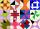Calculate the perimeter of isosceles triangle with arm length 73 cm and base length of 48 cm.
• Scalene triangleSolve the triangle: A = 50°, b = 13, c = 6
• SatinSanusha buys a piece of satin 2.4 m wide. The diagonal length of the fabric is 4m. What is the length of the piece of satin?
• Segment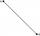Calculate the length of the segment AB, if the coordinates of the end vertices are A[10, -4] and B[5, 5].
• Triangle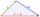Determine if it is possible to construct a triangle with sides 28 31 34 by calculation.
• Side cIn △ABC a=2, b=4 and ∠C=100°. Calculate length of the side c.
• TriangleProve whether you can construct a triangle ABC, if a=9 cm, b=6 cm, c=10 cm.
• Equilateral triangleCalculate the side of an equilateral triangle, if its area is 892 mm2.
• Diagonals of the rhombus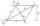Calculate height of rhombus whose diagonals are 12 cm and 19 cm.
• Length ITFind the length (circumference) of an isosceles trapezoid in which the length of the bases a,c and the height h are given: a = 8 cm c = 2 cm h = 4 cm
• Possible lengths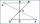Find the most possible lengths for the third side of a triangle with sides 20 and 18.
• 30-60-90The longer leg of a 30°-60°-90° triangle measures 5. What is the length of the shorter leg?
• Triangle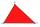For how many integer values of x can 16, 15 and x be the lengths of the sides of triangle?
• Distance problem 2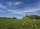A=(x,2x) B=(2x,1) Distance AB=√2, find value of x
• Medians and sides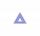Determine the size of a triangle KLM and the size of the medians in the triangle. K=(-5; -6), L=(7; -2), M=(5; 6).
• ThomasThomas lives 400 meters away from Samko, Robo from Thomas also 400 m and Samko from Robo 500. Anton lives 300 meters away from Robo further as Samko. How far away lives Anton from Rob?
• Square circles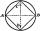Calculate the length of the described and inscribed circle to the square ABCD with a side of 5cm.
• Parallelogram perimeter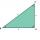The ABC triangle with sides a = 5cm, b = 3cm, c = 40mm has the center of the sides of the K,L,M. How many cm have the KBLM parallelogram perimeter?
• OctagonWe have a square with side 84 cm. We cut the corners to make his octagon. What will be the side of the octagon?
• ArmCalculate the length of the arm r of isosceles triangle ABC, with base |AB| = 14 cm and a height v=18 cm.

Do you have an interesting mathematical word problem that you can't solve it? Submit a math problem, and we can try to solve it.

We will send a solution to your e-mail address. Solved examples are also published here. Please enter the e-mail correctly and check whether you don't have a full mailbox.

Please do not submit problems from current active competitions such as Mathematical Olympiad, correspondence seminars etc...